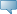# Lebanese American University WWW Information System

## Catalog Entries

Date
Dec 08, 2023Select the Course Number to get further detail on the course. Select the desired Schedule Type to find available classes for the course.

 MTH 309 - Graph Theory This course covers the fundamental concepts and methods of graph theory, and their applications in various areas of computing. Topics include graphs as models, representation of graphs, trees, distances, matching, connectivity, and flows in networks, graph colorings, Hamiltonian cycles, traveling salesman problem, and planarity. Course Learning Outcomes: 1) Students will be familiar with classical graph-theoretic terms. In particular, they will be able to define graphs, paths, cycles, trees, cliques, connectivity, and coloring. 2) Students will be able to prove classical results in graph theory. 3) Students will be able to understand algorithmic techniques for graph problems such as graph traversals, topological sorting, Euler formula and tours, minimum spanning trees, shortest path problems, and matching problems. 4) Students will be able to understand theorems and algorithms related to graph connectivity, coloring and planarity. 5) Students will be able to understand theorems and algorithms related to various forms of graph containment: minors, topological minors, etc… 6) Students will be able to understand theorems and algorithms related to Tree and Path decompositions, and the various graph metrics such as: treewidth, pathwidth, cutwidth, etc… 7) Students will be familiar with several NP-hard problems, such as Maximum Clique, Vertex Cover, Chromatic Number, Dominating Set, etc… 3.000 Credit hours 3.000 Lecture hours Levels: Undergraduate Schedule Types: Lecture, Tutorial Computer Science & Mathematics Department